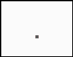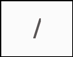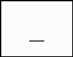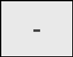# How do I input numbers?

### Basic input rules for whole numbers, fractions, mixed numbers, and decimals.

To enter numbers, you can use the number entry buttons, includingfor decimals andandfor fractions.

To enter negative values, put the number with thebutton in parentheses. Example: (-3), (-1/2), (-4_5/8), (-4.7).

##### Whole numbers.
To input whole numbers use the corresponding number entry buttons on the virtual keyboard.

##### Fractions.
Fractions are typed usingas the fraction bar.

So, "two-thirds" would be typed as 2/3.

"One-half" would be typed as 1/2.

Fractions can be entered with or without parentheses.

##### Mixed numberssymbol is used to separate the whole number and a fraction.

So, "three and one half" would be typed as 3_1/2.

##### Decimal numbers
Enter the number with the whole part separated from the decimal part by a decimal point (ex. 12.34, 0.4 or .4).

Monday, December 4, 2023

Contact